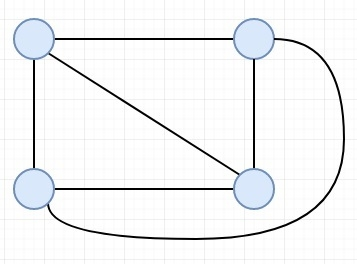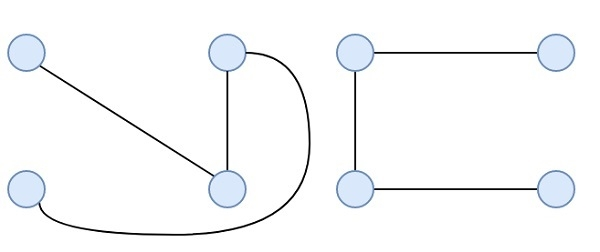# Maximum Possible Edge Disjoint Spanning Tree From a Complete Graph in C++

Suppose we have a complete graph; we have to count number of Edge Disjoint Spanning trees. The Edge Disjoint Spanning trees are spanning trees, where no two trees in the set have an edge in common. Suppose the N (number of vertices) is 4, then output will be 2. The complete graph using 4 vertices is like below −Two edge disjoint spanning trees are like −The maximum number of edge disjoint spanning tree from a complete graph, with N vertices will be $[\frac{n}{2}]$

## Example

#include <iostream>
#include <cmath>
using namespace std;
int maxEdgeDisjointSpanningTree(int n){
return floor(n/2);
}
int main() {
int n = 4;
cout << "Maximum Edge Disjoint Spanning Tree: " <<
maxEdgeDisjointSpanningTree(n);
}

## Output

Maximum Edge Disjoint Spanning Tree: 2